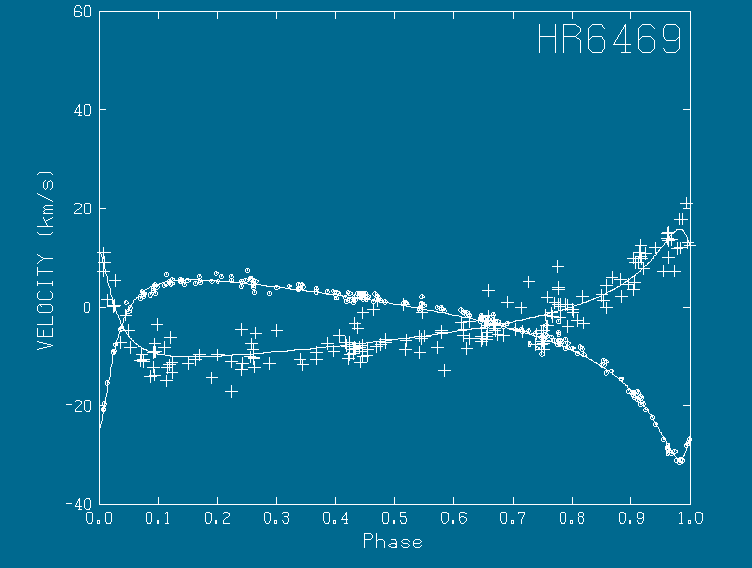## HR6469

This plot shows the radial velocities of the primary and secondary of the star system HR6469. This system is a triple star system, where the third star is a subcomponent of the secondary. The third star makes itself known by means of a reflex velocity in the secondary (the parent of the third star). The plot shows the velocity of the primary (circles) and the secondary (pluses) with the reflex velocity removed. The solution was obtained with the Binary program where speckle and spectroscopic data were simultaneously considered. The data were originally published by Scarfe, Barlow, Fekel, Rees, Lyons, Bolton, McAlister, and Hartkopf in the A. J., 107, 1529 (1994).

An example of the output (not the ultimate solution) is:

``` Period=                                         5.522380371     +-0.2420677E-02
Time of periastron passage=                     1986.371952     +-0.3332232E-02
Eccentricity=                                  0.6818927499     +-0.2190056E-02
Angle of line of nodes=                         321.5173704     +-0.3737605
Inclination=                                    55.93759981     +-0.4013246
Angle from node to periastron=                  222.8899259     +-0.3586578
Semimajor axis in "=                           0.4394817537E-01 +-0.3488334E-03
Ratio of semimajor axes (total to primary)=     1.711966236     +-0.8147726E-02
Parallax=                                      0.1487551549E-01 +-0.9626023E-04